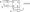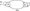# Logic Design December 2010

Note: Answer any FIVE full questions, selecting at least TWO questions from each part.

PART-A

1. Draw the logic circuit whose Boolean equation is Y = A + B + C , use only NAND gates.

b. Find the minimal sum and minimal product using Karnaugh map. f(a,b,c,d) = ^m(6,7,9,10,13) + d(l,4,5,ll)

c. Find the prime implicants for the following function using Quine Mccluskey      method: f(a,b,c, d) = m(l,2,8,9,l 0,12,13,14)

2 a. Implement the following function using a 8 : 1 multiplexer

f(a,b,c,d)~2]m(03lJ5,6,8,10,12,15)

b. Describe the working principle of a 3 : 8 decoder. Realize the following Boolean expressions using the 3 : 8 decoder : F, (A, B, C) = £ m(l,2,3,4) F2 (A, B, C) = £ m(3,5,7)           (06 Marks)

c. What is PLA? How does PLA differ from PAL?

d. Write HDL code for a 4 to 1 Mux considering any model.

3 a. How is 2’s complement representation used to perform subtraction? Give an example.

b. Show how two 7483 can be used to add/subtract two 8 bit numbers. Draw a neat diagram and explain its working.

c.   Design a 2 bit fast adder. Give its implementation using gates.

4 a. Calculate the clock cycle time for a system that uses a clock, that has a frequency of:

i)10 MHz                   ii) 6 MHz                  iii) 750 KHz

b. With a neat block diagram, explain the working of a Master-Slave JK flip flop. Also write its truth table.                                                                                                                                (07‘Marks)

c.   Explain the function of the circuit shown here with the state transition diagram.PART-B

5 a. Draw the logic diagram of a 4 bit serial in serial out shift register using JK flip flop and explain its working with an example.

b. Give the HDL code for a shift register of 5 bits constructed using D flip flops.

c. Construct a mod 8 asynchronous counter and write the truth table and draw waveforms.

d. Design a mod 4 synchronous counter using a -ve edge triggered JK flip flop. Draw the state transition diagram.                                                                                                      (06 Marks)

6 a. For the following state transition diagram, design equations for Moore model and generate the circuit diagram.Fig.Q6 (a)

b. Design an asynchronous sequential logic circuit for state transition diagram shown below: tot\0\ I o 1)

Fig.Q6 (b)

c.   How does state transition diagram of a Moore machine differ from Mealy machine?

7 a. Draw a binary ladder network for a digital input 1000 and obtain its equivalent circuit.

b.   Explain the concept of “successive approximation” of a A/D converter.

c. In a 8 bit counter type A/D converter driven by 500 KHz clock, find :

i) Conversion time

ii) Average conversion time

iii) Maximum conversion time.

8 a. Explain the working of CMOS NAND, NOR gates.

b. Explain with a neat diagram, working of a 2 input NAND gate TTL with

totempole output.

c. Explain how transistor acts as a switch. Define power dissipation and propagation delay time.